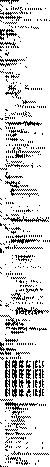Steven Pemberton : Views and Feelings

January 1996

# Programmers Are Humans Too

Look at this small C program to write the time on the terminal:

```#include <sys/types.h>
#include <sys/time.h>
main() {
struct timeval tp; struct timezone tzp;
struct tm *lt;
long secs1970;
gettimeofday(&tp, &tzp);
secs1970= tp.tv_sec;
lt = localtime(&secs1970);
printf("%d:%d:%d\n",
lt->tm_hour, lt->tm_min,  lt->tm_sec);
}```

A dozen lines of code, which will compile without change on any decent quality C compiler on any computer in the world.

I wrote this code, and I hereby donate it to the public domain.

Now consider the code below for an analogue clock (reduced to fit it on the page), a short example of the many examples of such clocks. A thousand plus lines of pure hell, catching events, and resizing windows, and goodness knows what, that will run on one computer only, for just one of the several windowing systems that run on that computer, which has not a single line in common with any of the other clock programs for that machine, and in fact has only a handful of lines in it that has anything to do with the concept of time at all. (And by the way, it's copyrighted code so don't go copying it!)In the middle ages, 1545 to be exact, the mathematician Cardan wrote in his Ars Magna (in Latin, thus my thanks to Lambert Meertens for this translation):

Raise the third part of the coefficient of the unknown to the cube, to which you add the square of half the coefficient of the equation, and take the root of the sum, namely the square one, and this you will copy, and to one {copy} you add the half of the coefficient that you have just multiplied by itself, from another {copy} you subtract the same half, and you will have the Binomium with its Apotome, next, when the cube root of the Apotome is subtracted from the cube root of its Binomium, the remainder that is left from this is the determined value of the unknown.

What he was trying to say was that one root of x3 + px = q is calculated so:

d = (p/3)3+(q/2)3
c = sqrt(d)
b = c + (q/2)
a = c - (q/2)
x = cuberoot(b) - cuberoot(a)

a calculation that any reasonably trained schoolchild can even prove nowadays.

One of the pieces of evidence for me that HCI hasn't yet reached maturity is the fact that it is so difficult to write a GUI program.

When we can agree on a programming interface to write GUI programs that run on all windowing systems, when we can program without being confronted with all sorts of low-level trivia, when our programs only represent the true functionality of the program, then we'll have a mature discipline!First published in the SIGCHI Bulletin, January 1996